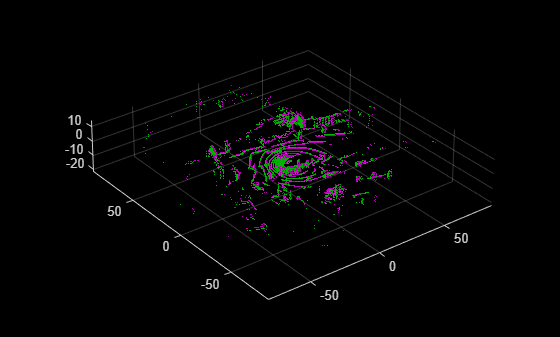# detectLOAMFeatures

Detect LOAM feature points from 3-D lidar data

Since R2022a

## Syntax

``points = detectLOAMFeatures(ptCloudOrg)``
``points = detectLOAMFeatures(ptCloudOrg,Name=Value)``

## Description

example

````points = detectLOAMFeatures(ptCloudOrg)` detects lidar odometry and mapping (LOAM) features in a point cloud based on curvature values. The function computes the curvature of each point using the closest neighbors of that point in the same laser scan. The curvature value of a feature point determines whether the function classifies it as a sharp edge, less sharp edge, planar surface, or less planar surface point.```
````points = detectLOAMFeatures(ptCloudOrg,Name=Value)` specifies options using one or more name-value arguments. For example, `NumRegionsPerLaser`=`6` sets the number of regions to split each laser scan to `6`. Unspecified arguments have default values.```

## Examples

collapse all

Load an organized lidar point cloud from a MAT file into the workspace.

```ld = load("drivingLidarPoints.mat"); ptCloudOrg = ld.ptCloud;```

Detect lidar odometry and mapping (LOAM) feature points.

`points = detectLOAMFeatures(ptCloudOrg);`

Visualize the LOAM points.

```figure show(points)```Read point cloud data from a Velodyne PCAP file into the workspace.

`veloReader = velodyneFileReader("lidarData_ConstructionRoad.pcap","HDL32E");`

Read the first point cloud from the data. Use this point cloud as the fixed point cloud.

`fixedPtCloud = readFrame(veloReader,1);`

Detect LOAM feature points in the fixed point cloud.

`fixedPoints = detectLOAMFeatures(fixedPtCloud);`

Downsample the less planar surface points from the fixed point cloud, to improve registration speed.

```gridStep = 1; fixedPoints = downsampleLessPlanar(fixedPoints,gridStep);```

Read and detect LOAM feature points from the fifth point cloud in the data. Use this point cloud as the moving point cloud.

```movingPtCloud = readFrame(veloReader,5); movingPoints = detectLOAMFeatures(movingPtCloud);```

Downsample the less planar surface points from the moving point cloud.

`movingPoints = downsampleLessPlanar(movingPoints,gridStep);`

Register the moving point cloud to the fixed point cloud.

`tform = pcregisterloam(movingPoints,fixedPoints);`

Transform the moving point cloud to align it to the fixed point cloud.

`alignedPtCloud = pctransform(movingPtCloud,tform);`

Visualize the aligned point clouds. Points from the fixed point cloud display as green, while points from the moving point cloud display as magenta.

```figure pcshowpair(alignedPtCloud,fixedPtCloud)````veloReader = velodyneFileReader("lidarData_ConstructionRoad.pcap","HDL32E");`

Read first and fifth point clouds.

```fixedPtCloud = readFrame(veloReader,1); movingPtCloud = readFrame(veloReader,5);```

Detect LOAM feature points.

```fixedPoints = detectLOAMFeatures(fixedPtCloud); movingPoints = detectLOAMFeatures(movingPtCloud);```

Create point cloud objects with the LOAM points.

```fixedPtCloudLoam = pointCloud(fixedPoints.Location); movingPtCloudLoam = pointCloud(movingPoints.Location);```

Register the point clouds.

`tform = pcregistericp(movingPtCloudLoam,fixedPtCloudLoam);`

Transform the moving point cloud to align it to the fixed point cloud.

`alignedPtCloud = pctransform(movingPtCloud,tform);`

Visualize the aligned point clouds.

```figure pcshowpair(alignedPtCloud,fixedPtCloud)```## Input Arguments

collapse all

Organized point cloud, specified as a `pointCloud` object. The point cloud object must contain an organized point cloud with a `Location` property of size M-by-N-by-3 matrix, where M is the number of laser scans and N is the number of points per scan. The first page represents the x-coordinates, the second page represents the y-coordinates, and the third page represents the z- coordinates for each point.

### Name-Value Arguments

Specify optional pairs of arguments as `Name1=Value1,...,NameN=ValueN`, where `Name` is the argument name and `Value` is the corresponding value. Name-value arguments must appear after other arguments, but the order of the pairs does not matter.

Example: `detectLOAMFeatures(ptCloudOrg,NumRegionsPerLaser = 6)` sets the number of regions to split each laser scan into for feature point detection to `6`.

Number of regions per laser scan for feature point detection, specified as a positive integer. The function splits each laser scan into regions that contain equal numbers of points. The algorithm detects up to the maximum number of points for each feature type in each region. You can specify the maximum number of sharp edge, less sharp edge, and planar surface points by using the `MaxSharpEdgePoints`, `MaxLessSharpEdgePoints`, and `MaxPlanarSurfacePoints` arguments, respectively. Increase the number of spatial regions to detect more edge points and planar surface points uniformly throughout the point cloud. Detecting more edge points and planar surface points can improve registration accuracy using LOAM points, but can decrease the processing speed of the function.

Maximum number of sharp edge points per laser scan region, specified as a positive integer. The sharp edge points are the points with the highest curvatures.

Maximum number of less sharp edge points per laser scan region, specified as a positive integer. The less sharp edge points are the points with the highest curvature values after the sharp edge points.

Maximum number of planar surface points per laser scan region, specified as a positive integer. The planar surface points are the points with the lowest curvature values.

## Output Arguments

collapse all

LOAM feature points, returned as a `LOAMPoints` object.

## Tips

• Because LOAM feature point detection supports only organized point clouds, convert an unorganized point cloud into an organized point cloud by using the `pcorganize` function.

• The LOAM algorithm relies on the neighborhood of each point to compute its curvature and identify which points are on the boundaries of occluded regions. These points are considered unreliable points. Because of this unreliability, any preprocessing steps to the point clouds prior to feature point detection is not recommended.

• You can increase registration accuracy by increasing the maximum total number of feature points the function can detect. To increase the total number of feature points, increase the value of one or more of the `MaxSharpEdgePoints`, `MaxLessSharpEdgePoints`, and `MaxPlanarSurfacePoints` arguments. Note that this can also decrease the processing speed.

## Algorithms

• The feature point detection algorithm supports VLP-16, HDL-32, and other spinning lidar sensors also known as surround sensors.

• The laser ID of each point corresponds to the laser that detects the point. For organized point clouds used with this algorithm, the `pointCloud` `Location` property stores the collected points as an M-by-N-by-3 matrix. Each row M represents a separate laser scan with N number of points, and 3 represents the x,y,z coordinates for each point.

• The algorithm uses the laser ID for point detection in `detectLOAMFeatures` and for point matching in `pcregisterloam`.

 Zhang, Ji, and Sanjiv Singh. “LOAM: Lidar Odometry and Mapping in Real-Time.” In Robotics: Science and Systems X. Robotics: Science and Systems Foundation, 2014. https://doi.org/10.15607/RSS.2014.X.007.